# Excel Applications for Accounting Principles

## Quiz 18 : Cost-Volume-Profit Analysis CVPLooking for Accounting Homework Help?

## Quiz 18 :Cost-Volume-Profit Analysis CVP

Question TypePROBLEM DATA Poleski Manufacturing, which maintains the same level of inventory at the end of each year, provided the following information about expenses anticipated for next year: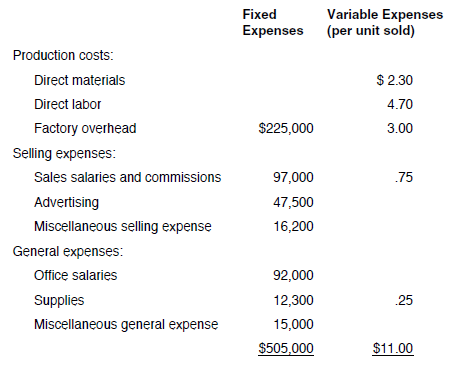The selling price of Poleski's single product is $16. In recent years, profits have fallen and Poleski's management is now considering a number of alternatives. Poleski wants to have a net income next year of$250,000, but expects to sell only 120,000 units unless some changes are made. REQUIREMENT The president of Poleski has asked you to calculate the company's projected net income (assuming 120,000 units are sold) and the sales needed to achieve the company's net income objective for next year. Also, compute Poleski's contribution margin per unit, contribution margin ratio, and break-even point for next year. The worksheet CVP has been provided to assist you. Note that the data from the problem have already been entered into the Data Section of the worksheet.
Free
Essay

Cost volume profit analysis (CVP analysis):
This analysis depends on the breakeven point determined to make short term decisions. This is useful for the managers in making decisions regarding the number of units to be produced and sold to earn the target profit.
Compute the requirements in the excel sheet as shown below: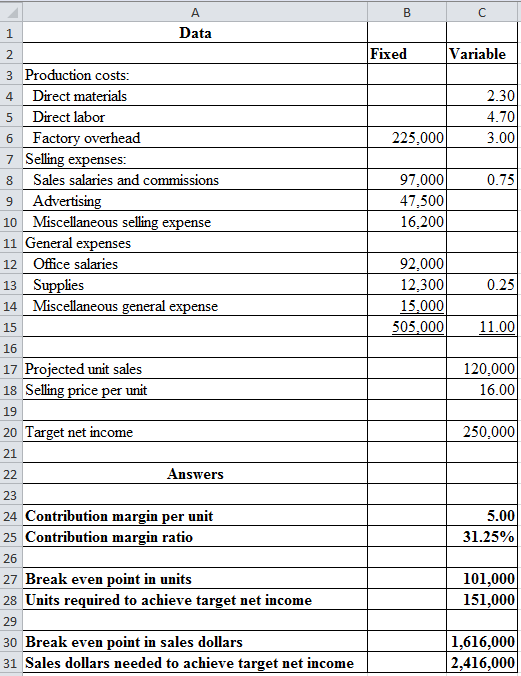The formulas to compute the requirements are shown below: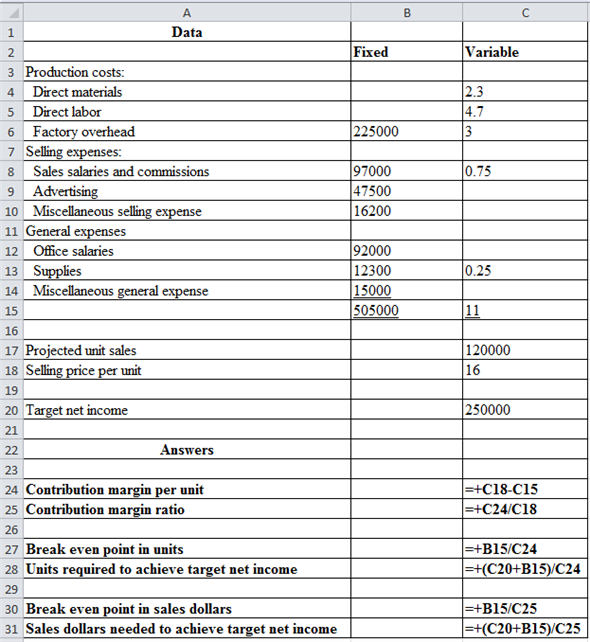Explanation of terms:
• Contribution refers to the balance of sales revenue left after incurring the variable costs. Contribution per unit is expressed in terms of amount per unit whereas contribution margin ratio is expressed in terms of percentage of sales.
• Breakeven point refers to a point of sale where the fixed cost is equal to the contribution. Therefore, the entity will not earn any profit nor incur any loss.

To earn the target net income the entity should also earn the target profit in addition to the fixed cost. The fixed cost added to the target profit gives the target contribution.

TagsPROBLEM DATA Poleski Manufacturing, which maintains the same level of inventory at the end of each year, provided the following information about expenses anticipated for next year:The selling price of Poleski's single product is $16. In recent years, profits have fallen and Poleski's management is now considering a number of alternatives. Poleski wants to have a net income next year of$250,000, but expects to sell only 120,000 units unless some changes are made. REQUIREMENT Open the file CVP from the website for this book at cengagebrain.com. Enter the formulas where indicated on the worksheet. Enter your name in cell A1. Save the solution as CVP2 and print the worksheet. Also print your formulas. Check figures: Break-even point in sales dollars (cell C35), $1,616,000; Net income (cell C38),$95,000.
Free
Essay

Open the file indicated in question. Enter formulas in the worksheet using cell references. After entering required formulas results will be visible. Obtained results are shown in image below.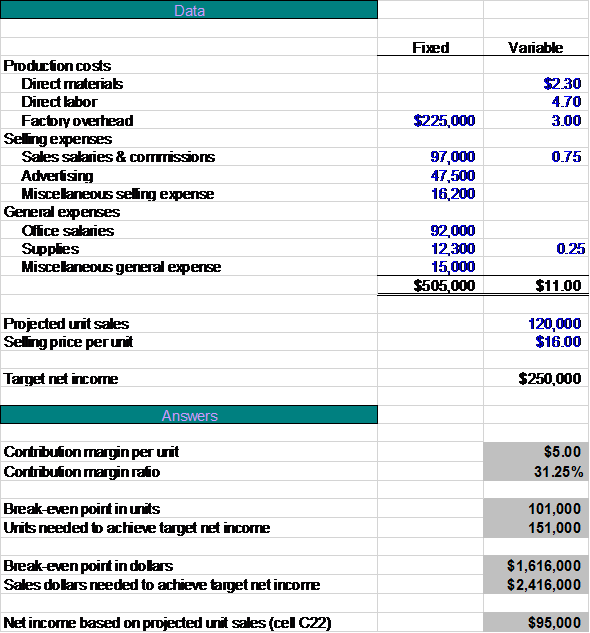Formulas used are stated below.
The contribution margin per unit is calculated with the help formula given below.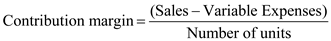The contribution margin ratio is calculated with the help formula given below.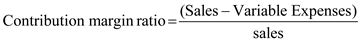The break-even point in units is calculated with the help formula given below.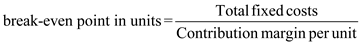The break-even point in dollars is calculated with the help formula given below.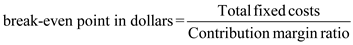Number of units needed to achieve target income is calculated with the help formula given below. Idle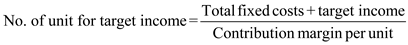Sales dollars needed to achieve target net income is calculated with the help formula given below.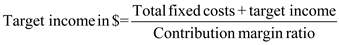Net income is calculated with the help formula given below.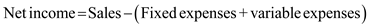TagsPROBLEM DATA Poleski Manufacturing, which maintains the same level of inventory at the end of each year, provided the following information about expenses anticipated for next year:The selling price of Poleski's single product is $16. In recent years, profits have fallen and Poleski's management is now considering a number of alternatives. Poleski wants to have a net income next year of$250,000, but expects to sell only 120,000 units unless some changes are made. REQUIREMENT Based on Poleski's current situation, will it earn its target net income If not, how many units need to be sold to achieve the target Explain.
Free
Essay

Based on the following data, Company P will not earn its target net income of $250,000. Based on the projected unit sales, its net income will be$95,000 only.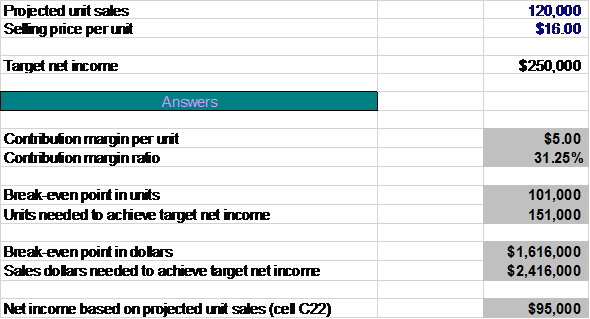Hence, the net income is $95,000 , lesser than target income. In order to achieve target net income, Company P needs to sell no less than 151,000 units at the$16 per unit selling price. That figure can be determined by entering different numbers into the cell for projected unit sales (C22) until net income based on projected unit sales (cell C38) is equal to $250,000.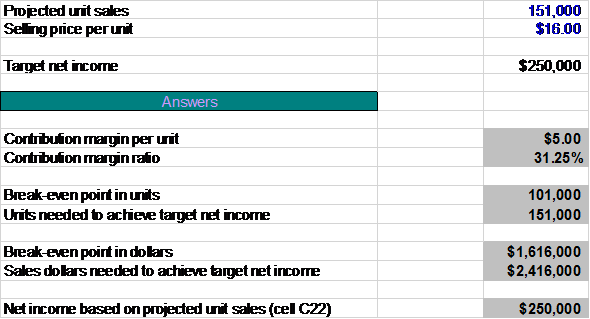Hence, the net income is$250,000.

TagsWHAT-IF ANALYSIS The president of Poleski would like to know the effect that each of the following suggestions for improving performance would have on contribution margin per unit, sales needed to break even, and projected net income for next year. Each change should be considered independently. Reset the Data Section to its original values after each suggestion is analyzed. Fill in the table following the suggestions with the results of your analysis. a. The president suggests cutting the product's price. Since the market is relatively sensitive to price, "... a 10% cut in price ought to generate a 30% increase in sales (to 156,000 units). How can you lose " b. The sales manager feels that putting all sales personnel on straight commission would help. This would eliminate $77,000 in fixed sales salaries expense. Variable sales commissions would increase to$2.00 per unit. This move would also increase sales volume by 30%. c. Poleski's head of product engineering wants to redesign the package for the product. This will cut $1.00 per unit from direct materials and$0.50 per unit from direct labor, but will increase fixed factory overhead by $100,000 for additional depreciation on the new packaging machine. The package redesign would not affect sales volume. d. The firm's consumer marketing manager suggests undertaking a new advertising campaign on Facebook. This would cost$30,000 more than is currently planned for advertising but would be expected to increase sales volume by 30%. e. The production superintendent suggests raising quality and raising price. This will increase direct materials by $1.00 per unit, direct labor by$0.50 per unit, and fixed factory overhead by $110,000. With improved quality, "... raise the price to$18.50 and advertise the heck out of it. If you double your current planned advertising, I'll bet you can increase your sales volume by 30%."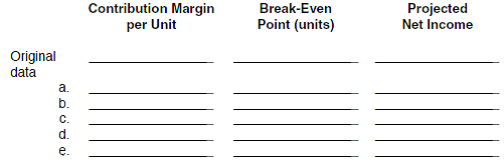Essay
TagsWHAT-IF ANALYSIS From this analysis, which suggestion would you advise Poleski Manufacturing to try Explain.
EssayWHAT-IF ANALYSIS The president of Poleski can't figure out where her math went wrong. Use the CVP2 file to determine how many units would have to be sold at $14.40 in order for the firm to achieve its target net income of$250,000. Explain how the president's analysis is incorrect.